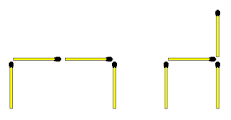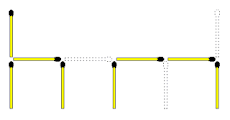## Drop down Tab menu for Site

### FIGURE OUT PUZZLES - matchstick puzzles (03)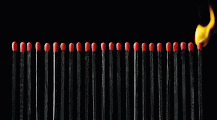QUESTION 1
Move only 1 matchstick to form a square.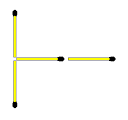The arrow shows where the square is formed.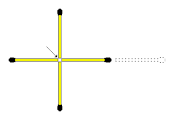QUESTION 2
Move 3 matchsticks to show how this house would look like from a different angle.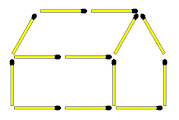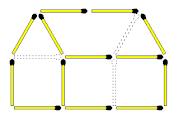QUESTION 3
Shown below are 2 glasses and 2 crosses. The distance between A and B is exactly the length of one matchstick.
Move 4 matchsticks to place the crosses inside the 2 glasses. You are not allowed to move the crosses.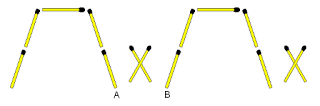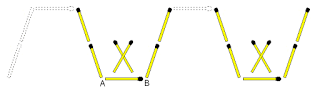QUESTION 4
In total, how many different routes are there from point A to point J? You can only move in the direction of the matchstick heads.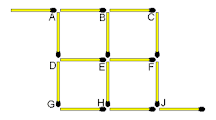There are 6 routes:
ABCFJ
ABEFJ
ABEHJ

QUESTION 5
Move 4 matchsticks to form a cross.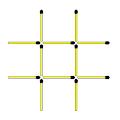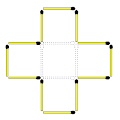QUESTION 6
Move 2 matchsticks to make the statement true.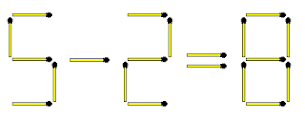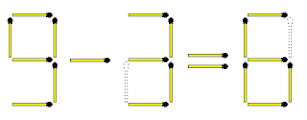QUESTION 7
Move only 1 matchstick to make the equation true.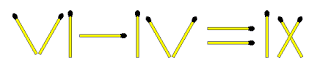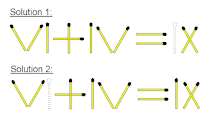QUESTION 8
Figure 1 above shows a garden spade and fork. In Figure 2 the two garden tools are placed next to each other.
The challenge is to swap positions in Figure 2 by moving only 4 matchsticks. In your solution the spade must still point downwards and the fork still upwards.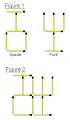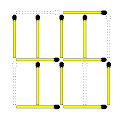QUESTION 9
The triangles form a sequence from 1 to 3. What should the structure look like in position 4?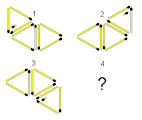Triangles A and B remain in the same position. Triangle C moves clockwise rotating itself around the corner of the next triangle as indicated. The broken matchstick remains unchanged within triangle C and moves along as triangle C rotates.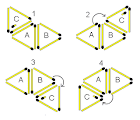QUESTION 10
Move 2 matchsticks to make the equation true.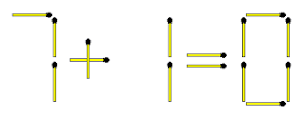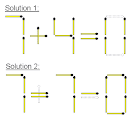QUESTION 11
Move 2 matchsticks to make the equation true.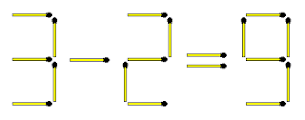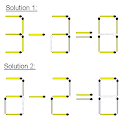QUESTION 12
The time on a digital clock is 6H27 am as shown above. Move 3 matchsticks to show the time 2 hours and 34 minutes later.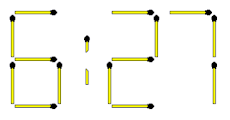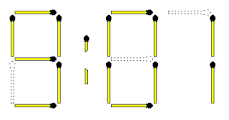QUESTION 13
Create a circle by rearranging the 8 matchsticks above. All the matchsticks must be flat on the surface.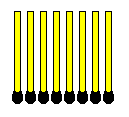The circle is in the middle.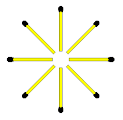QUESTION 14
The 2 arrows below are pointing in the West direction. Move 4 matchsticks to make it point in a South-West direction.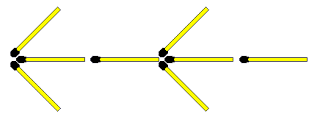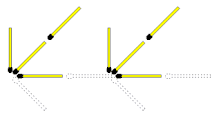QUESTION 15
Move only 1 matchstick to create 3 squares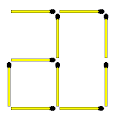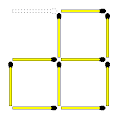QUESTION 16
Move only 1 matchstick to make the equation true.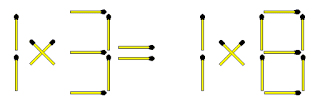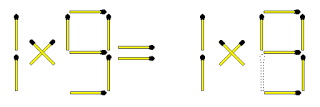QUESTION 17
Move only 1 matchstick to make the equation true.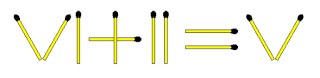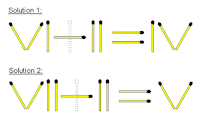QUESTION 18
Which one of the 4 structures below, is the odd one out?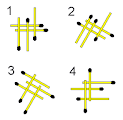Two rectangles are formed in each structure, except in number 3.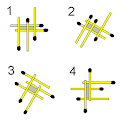QUESTION 19
The equation is not true. TAKE AWAY 4 matchsticks to make it true.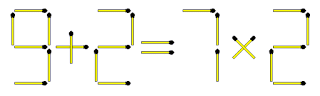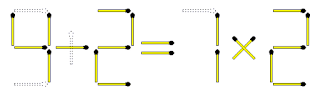QUESTION 20
Move the chair to the left of the table by moving only 3 matchsticks. The distance between the table and the chair is exactly the length of one matchstick.